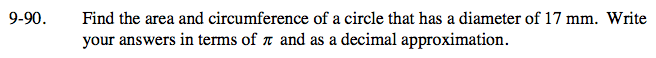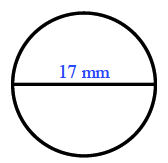Home > CC2 > Chapter 9 > Lesson 9.2.4 > Problem9-90

9-90.

Find the area and circumference of a circle that has a diameter of 17 mm. Write your answers in terms of π and as a decimal approximation. Homework Help ✎Use the formulas for the area and circumference of a circle:

Area: π • r 2

Circumference: π · 2r or π · dArea = π (8.5)2 = 72.25π ≈ 226.98 mm2